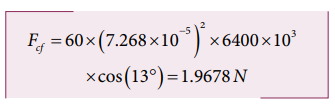Home | | Physics 11th std | Centrifugal Force due to Rotation of the Earth

# Centrifugal Force due to Rotation of the Earth

Even though Earth is treated as an inertial frame, it is actually not so.

Centrifugal Force due to Rotation of the Earth

Even though Earth is treated as an inertial frame, it is actually not so. Earth spins about its own axis with an angular velocity ω. Any object on the surface of Earth (rotational frame) experiences a centrifugal force. The centrifugal force appears to act exactly in opposite direction from the axis of rotation. It is shown in the Figure 3.48.

The centrifugal force on a man standing on the surface of the Earth is Fc = mω2r

where r is perpendicular distance of the man from the axis of rotation. By using right angle triangle as shown in the Figure 3.48, the distance r = R cos θ

Here R = radius of the Earth

and θ = latitude of the Earth where the man is standing.## Solved Example Problems for Centrifugal Force due to Rotation of the Earth

### Example 3.26

Calculate the centrifugal force experienced by a man of 60 kg standing at Chennai? (Given: Latitude of Chennai is 13°

### Solution

The  centrifugal force is given by Fc = mω2 R cosθ

The angular velocity (ω) of Earth = 2π/T.

where T is time period of the Earth (24 hours)The radius of the Earth R = 6400 Km = 6400 × 103 m

Latitude of Chennai =13°A 60 kg man experiences centrifugal force of approximately 2 Newton. But due to Earth’s gravity a man of 60 kg experiences a force =mg = 60 × 9.8 = 588N. This force is very much larger than the centrifugal force.

Study Material, Lecturing Notes, Assignment, Reference, Wiki description explanation, brief detail
11th Physics : UNIT 3 : Laws of Motion : Centrifugal Force due to Rotation of the Earth |